## Friday, May 29, 2015

### My First Kindle Book is Uploaded and Processing

Urged on by colleagues and bolstered by the number of hits I'm getting for all of my Regents exam blog posts in these days leading up to the next round of testing, I decided to collect the Algebra 1 (Common Core) tests together under in one volume, along with my answers and explanations.

It's a first try, and it may not go as well as I would have liked. I will be honest: I pushed this as hard and fast as I could, while still taking time to review it for errors. However, I had a deadline: the test is coming up in a couple of weeks. If I don't have this up now, I miss an important window of opportunity.

I have read up on Kindle publishing in the past. I have read how to convert a Word file, and I've read that the easiest thing to do is to post a .PDF. I opted for the .PDF because of the extra care that needed to be taken with all the images in Word files. There are a lot of images in these tests.

That being said, Kindle gave me a warning about Bad Things that might happen converting a PDF. From the preview, I think that might've occurred. I thought that a PDF file would be better for educators -- I may have been wrong about that.

It's a little early yet. I'll know soon enough. And changes can always be made at a later time and uploaded.

Time will tell.

## Wednesday, May 27, 2015

### Flipped

(Click on the comic if you can't see the full image.)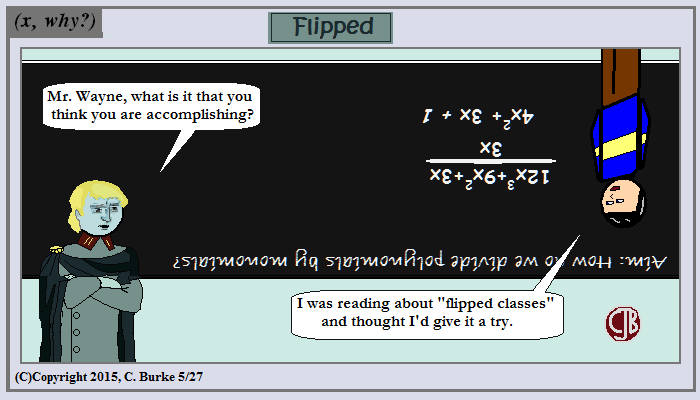I'm sure you always expected he'd be the first one to flip.

This comic is long overdue, given how long ago I was introduced to the concept of the flipped classroom by (x, why?) reader, and occasional comic user, Stacey Roshan. Here is a Washington Post story from 2012 her flipped classroom.

You do realize (and she probably does, as well) that I'm practically daring her to use this comic in a future lesson.

Come back often for more funny math and geeky comics.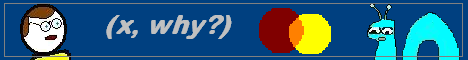## Monday, May 25, 2015

### Memorial Day Bee-B-Q

(Click on the comic if you can't see the full image.)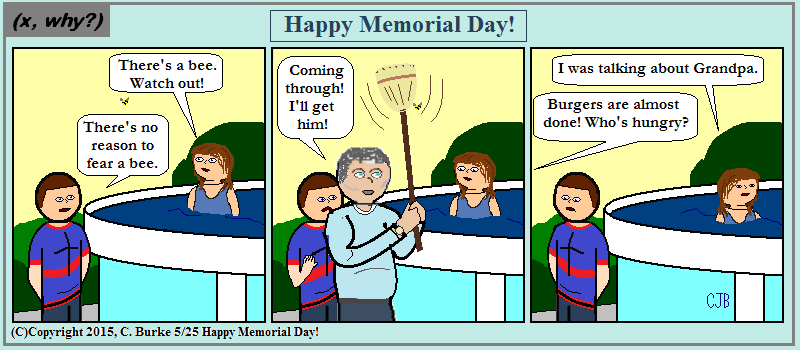He's a 1-Bee Bomber, or a 1-Bee Broomer.

Some things never change: like getting the Ol' Man away from the grill for more than a few seconds.

And while you're grilling and enjoying the day off, keep in mind why you have the day off and the freedom to grill whatever you want in peace. Well, except for the bees.

But even then, there's someone who's keeping you safe.

Come back often for more funny math and geeky comics.## Sunday, May 24, 2015

### Regents: Residuals and the Line of Best Fit (August 2014 Common Core Algebra 1)

Update: I now have a Common Core Regents Review books available on Amazon.

New York State Common Core Algebra 1 Regents Exam, August 2014.

31. The table below represents the residuals for a line of best fit. Plot these residuals on the set of axes below.

Using the plot, assess the fit of the line for these residuals and justify your answer.

This is another new topic in Algebra 1. The residuals for a line of best fit are distance a point is away from the line. It is the actual value – the expected value. In other words, subtract the y-value of the data point from the y-value of the trend line for that x-value. This could give you a negative value; that’s okay.

A trend line should go through the middle of the data on a scatter plot. That means that some values are above and some values are below the line. Therefore, there should be many positive and negative residuals. If the residual points are plotted, they should be scattered out in such a way that there is no pattern (almost like a scatter plot with no correlation). If there is a pattern, then there was a mistake.

If the pattern for the residuals is somewhat linear, there was a mistake calculating the line of best fit.

If the pattern for the residuals is a curve (such as a parabola), then it was incorrect to try to use a linear regression in the first place, instead of, say, a quadratic or some other regression.

When the residuals are plotted, you will get the graph below. As you can see, it is a poor fit because there is a pattern.

To get both points for this question, you needed a correct plot, stated that it is a poor fit, and gave a correct justification for the poor fit (a pattern was formed). One mistake or one thing missing would cost you one point point. More than one mistake would result in zero points for this question.

## Friday, May 22, 2015

### Three and Four

(Click on the comic if you can't see the full image.)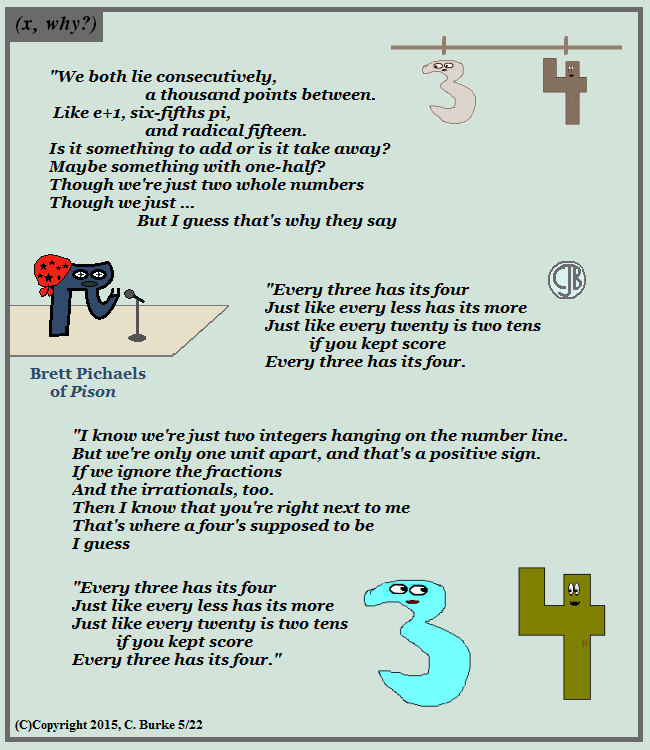My other choice: Every Kirk has his Gorn . . .

The more I think about it, I might've gotten enough sci-fi references to fill that in, had I tried, had I tried ... Damn it, Jim!

Meanwhile, 80s Week continues.

Come back often for more funny math and geeky comics.## Tuesday, May 19, 2015

So first a thanks to Gregory Taylor (@mathtans) of Canada -- I'll get you for this, even as five other educators are preparing to get me -- for including me in his list of five “Twitter Stars”, following his own nomination by Chris Smith (@aap03102) in Scotland. I'm in NYC, so we've crossed the pond and come down across the border, eh?

A little more about Greg: We first met when he stumbled across my webcomic, and he let me know about a project of his own, Taylor's Polynomials, which was something new. Not quite a comic, not quite a story serial. I admit, I didn't always keep up to date with it, but I liked the song parodies. ("Making a Graph! Making a Graph!") And, as noted above, he has a regular blog as well.

Greg has a very detailed post about the beginnings of the #TwitteratiChallenge hashtag, so I don't need to, er, rehash it. But I will repeat his link to the Teacher Toolkit post, explaining all of this.

So keeping it fun and without getting too introspective about this, I'll move along and play by the rules, sort of, maybe ...INTRO: In the spirit of social-media-educator friendships, this summer it is time to recognize your most supportive colleagues in a simple blogpost shout-out. Whatever your reason, these 5 educators should be your 5 go-to people in times of challenge and critique, or for verification and support.

#### RULES

There are only 3 rules.
1. You cannot knowingly include someone you work with in real life.
3. You will need to copy and paste the title of this blogpost, the rules, and what to do information into your own blog post.

#### WHAT TO DO

If you would like to participate with your own list, here’s how:
1. Within 7 days of being nominated by somebody else, you need to identify colleagues that you rely on, or go to for support and challenge.
2. You need to write your own #TwitteratiChallenge blogpost. (If you do not have your own blog, try @StaffRm.)
3. As the educator nominated, that means that you reading this must either: a) record a video of themselves in continuous footage and announce their acceptance of the challenge, following by a pouring of your (chosen) drink over a glass of ice.
4. Then, the drink is to be lifted with a ‘cheers’ before the participant nominates their five other educators to participate in the challenge.
5. The educator that is now newly nominated has 7 days to compose their own #TwitteratiChallenge blogpost (use the hashtag) and identify who their top 5 go-to educators are.

I'll cheat a little on 3 & 4, because the beverage of choice would likely be hot, and it'd be a waste to pour it over ice. Even to make a concoction such as the much-talked-about "iced coffee" would require steps that would be too boring to watch. And not every beverage goes with ice anyway. But for the sake of the rules, I suppose that this is some part of that whole "ice challenge video" meme leftover from last summer. But I digress.

Coming up with five was a little difficult. First, I had to scratch a few off because they were nominated by Taylor (or along with him). Then I wanted to be a little fair to others: there are some people who will be in the lists of one of those whom I've nominated. Why make it harder for them? And then I realized I wouldn't have as much trouble as I thought getting up to five ... it'd be getting down to five.

In no particular order, if you believe that a math teacher couldn't find some "order" to justify any combination or permutation:

Stacey Roshan (@buddyxo): I think I've "known" Stacey longer than anyone in this post, with the exception of the aforementioned-soon-to-be-gotten Mr. Taylor. I use "known" in the Internet sense of "we don't actually know each other, but we've had long distance communication". Stacey contacted me about using one of my comics in a presentation, which is something we're all supposed to do -- ask permission, not use my comics, but, yeah, that, too. But it's when I started following her on Twitter that things got interesting. Stacey is a champion of the flipped classroom, a concept which intrigues me, but one that I probably couldn't have gotten past my Assistant Principal a couple years ago. There would be implementation issues for the population I was teaching, but I think it would be a great thing if they gave it a real chance. I know this because I follow what she's doing even if it doesn't apply to me right now.

Doug Robertson (@TheWeirdTeacher): Doug is the Weird Teacher. You know this because it follows the "@" symbol. And because it's written on his profile pic. Which is taken from the cover of his book. Which is so popular amongst teachers that it has its own weekly Book Club chat, as well as, not one, but *TWO* -- I'm a math teacher, I love to count, ah, ah, ah -- #WeirdEd chats each week. They're actually the same chat, at 7 pm PST and 7 pm EST, the latter for the East Coast teachers who find 10pm to be past their bedtimes, but who have managed to eat dinner by 7pm. (I seem to have one conflict or the other, but I make it when I can.) It's always a lively, fun discussion, and his weird sense of humor shines through every other day of the week in whatever random post he's, um, posting. If you think my humor is weird, remember that he has it in writing. So, Doug, I list you among the Top Five. (You're Welcome).

Michael Pershan (@mpershan): Michael may have the distinction of being the one person on this list that I've met. We were both at the NYC Math Teacher tweetup back in December 2014, with a bunch of other wonderful math people (a phrase that English teachers call "redundant"). Michael is a Geometry teacher, like I am. However, he teachers 3rd and 4th graders, which I don't -- I have HS Geometry. And yet, when he posts, I recognize the same of the (teaching) problems and situations and solutions and relate to them. Excluding my witty comments, I think I've replied to more of his posts with than any other teacher's, outside of chats. (But if you do exclude my witty comments, you're excluding 90% of my comments, for varying values of "witty".) Sidenote: the tweet-up was fun -- we need to do that again some time. I could use a night out.

Rosy Burke (@rosy_burke): I'm going to tell you two secrets about my little cousin: First, she's 238 years old in dog years. That's not actually a secret: he tweeted that one of her students came up with that. Doesn't look a day over 237, if you ask me, right? Second, she's not my cousin. That just came up in a chat, and we went with it. No one questioned it. Rosy is another teacher of younger grades, but that doesn't mean that I can't apply her tweets to an older audience. And, hopefully, with teachers like Rosy in the younger grades, I'll have more ready, more capable students in the upper grades. (You know, if they move across the country and just happen to find my school, whichever one that is.)

So that's my list, and now that I'm done, I realize that it could've been a lot longer.

And here's some other stuff that I'm including because Greg did, and I'm stealing it without asking permission. Shame on me, but I know he doesn't mind. (And I've already linked to his post.) Now, for the purists, the backtrack blogs:
-To me, from Gregory Taylor@mathtans
Via @aap03102
Via @mathsjem,
via @aegilopoides
via @KDWScience,
via @Chocotzar,
via @heatherleatt,
via @MaryMyatt,
via @cherrylkd, From SOURCE (TeacherToolkit, above).

You can also find @Sue_Cowley’s May 11th compilation here, and I've seen @JillBerry102 often pop up in association with the hashtag.

## Monday, May 18, 2015

### The Distance Around

(Click on the comic if you can't see the full image.)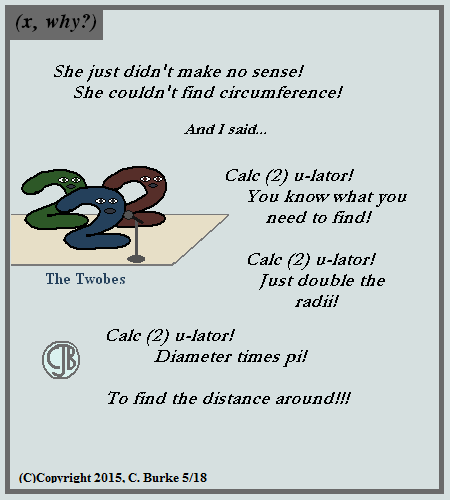If you quiz them, then you'll test Twobes ...

A little music trivia: this is the kind of song that I enjoy on the radio, but never bought the entire album (which is what they had back then). It's because of this that even though I've heard the song Talk to You Later many, many times over the (multiple periods of ten) years, it wasn't until about a week ago that I actually knew who sung the song. I might've had a couple of guesses, but they would've been wrong because I don't remember ever hearing the name The Tubes before.

The Tubes, and this song, have an interesting place in music history. A long time ago, in the early days of MTV -- you know, back when they played Music -- the network didn't air in places like NY or LA. It did, however, air on cable TV in places like Tulsa, OK. By co-incidence, Talk to You Later sold out in Tulsa despite not getting any airplay at all on the radio. MTV took full credit for this and went to music studios and told them that they needed to produce videos to advertise their records ... and give them to MTV.

The rest is History. As in thing that happened in the past because no one makes albums any more, MTV doesn't play music, and videos go straight to YouTube these days. (Or whatever the next thing after that is.)

Come back often for more funny math and geeky comics.## Friday, May 15, 2015

### Participation Rubric

(Click on the comic if you can't see the full image.)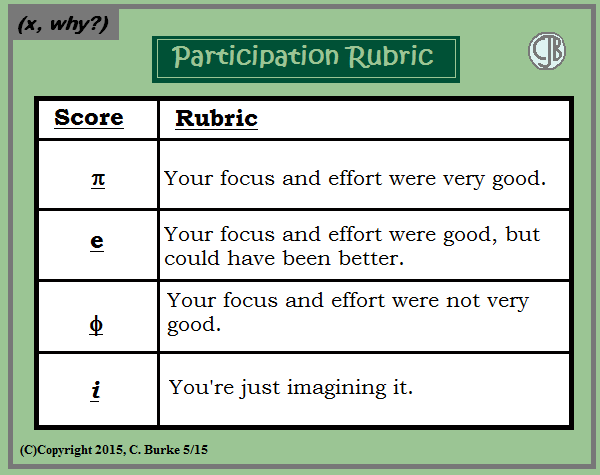Phi points is a lot better than epsilon points. So take the grade and cut your losses!

I think Ken would more likely have this hanging in his room than Mike.

Come back often for more funny math and geeky comics.## Thursday, May 14, 2015

### (x, why?) Mini: Irregular

(Click on the comic if you can't see the full image.)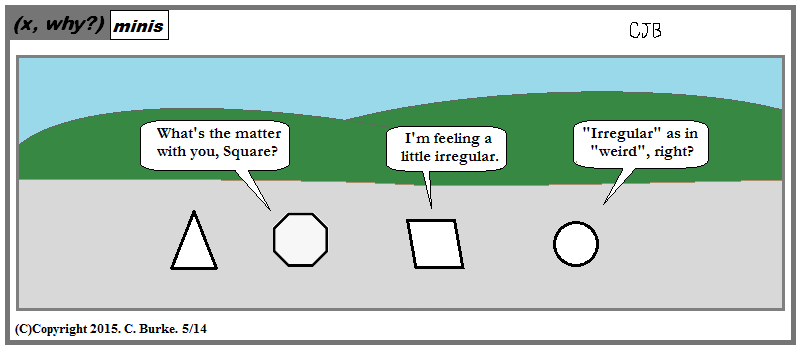His rhombustism is acting up again.

This was an alternate setup I originally had for what became this this comic instead.

But why waste a good setup, right? Right angle?

Come back often for more funny math and geeky comics.## Wednesday, May 13, 2015

### January 2015 Common Core Algebra Regents, Parts III & IV

Update: I now have a Common Core Regents Review books available on Amazon.

If you missed the previous post, here is Part I, and here is most of Part II.

The rest of the Common Core exam follows. It contains the last question from Part II and all of Parts III and IV.

32. Write an exponential equation for the graph shown below.

Explain how you determined the equation.

Exponential equations are in the form y = a(b)x. There are two methods to get the correct equation.
First: Label the points that we know, not the ones you have to guess at.
These are (2, 1), (3, 2), (4, 4), (5, 8). We can see that as x increases by 1, the value of y doubles. So the base (b) is 2. This gives us y = a(2)x. Plug in any (x, y) and solve for a, which is ¼ or .25. Either is acceptable. The answer is y = .25(2)x.

Second: put those same points into two LISTs on your graphing calculator. L1 = {2, 3, 4, 5}. L2 = {1, 2, 4, 8}. In the CALC menu, select ExpReg, for Exponential Regression. It will give you the same answer. EXPLAIN on your paper that you used the calculator and what steps you took (as I just did), and you will receive full credit. If you give the equation and just say “The calculator said so” then you will NOT get full credit.

### Part III – Four points each

33. Jacob and Zachary go to the movie theater and purchase refreshments for their friends. Jacob spends a total of \$18.25 on two bags of popcorn and three drinks. Zachary spends a total of \$27.50 for four bags of popcorn and two drinks.

Write a system of equations that can be used to find the price of one bag of popcorn and the price of one drink.

Using these equations, determine and state the price of a bag of popcorn and the price of a drink, to the nearest cent.

Define your variables: p = cost of 1 popcorn, d = cost of 1 drink
Write an equation for each purchase:
2p + 3d = 18.25
4p + 2d = 27.50.

If we double the first equation, we can use the Elimination Method to solve this System of Equations.
4p + 6d = 36.50
4p + 2d = 27.50 (subtract the second equation from the first)
4d = 9.00
d = 2.25. The price of a drink is \$2.25. Substitute in an original equation.
4p + 2(2.25) = 27.50
4p + 4.50 = 27.50
4p = 23.00
p = 5.75. The price of a popcorn is \$5.75.

34. The graph of an inequality is shown below.

a) Write the inequality represented by the graph.

b) On the same set of axes, graph the inequality x + 2y < 4.

c) The two inequalities graphed on the set of axes form a system. Oscar thinks that the point (2,1) is in the solution set for this system of inequalities. Determine and state whether you agree with Oscar. Explain your reasoning.

a. The y-intercept of the graph is -3. The slope is +2 (2 up, 1 over). The line is solid, not broken and it is shaded above the line, which means > (greater than or equal to).
y > 2x – 3.

b. If you don’t know how to graph an inequality is Standard Form, you can rewrite it in Slope-Intercept Form, which is y < – ½ x + 2. The graph will look like this:

c. Oscar is incorrect because the (2, 1) is on a broken line. Points on the broken line are NOT part of the solution. They are a boundary to the solution. Everything up to, but NOT including, the line is a solution. NOTE: Your answer to this question depends upon the work you did before it. If you made an earlier mistake, then part c has to be consistent with that mistake.

35. A nutritionist collected information about different brands of beef hot dogs. She made a table showing the number of Calories and the amount of sodium in each hot dog.

a) Write the correlation coefficient for the line of best fit. Round your answer to the nearest hundredth.

b) Explain what the correlation coefficient suggests in the context of this problem.

The only way to do this is with your graphing calculator. Put the first column in L1. Put the second column in L2. In the CALC menu, select LinearReg. You will get the equation of the trend line along with the correlation coefficient, which is always a number between -1 and 1.

The correlation coefficient, rounded to two decimal places, is 0.94.

This means that there is a strong positive correlation between the two sets of data.

36. a) Given the function f(x) = -x2 + 8x + 9, state whether the vertex represents a maximum or minimum point for the function. Explain your answer.

b) Rewrite f(x) in vertex form by completing the square.

Because the coefficient of x2 is negative, the parabola opens downward, which means that the graph has a top or a maximum point. (It goes down forever, so there is no bottom, no minimum.)

Complete the square as follows: Subtract 9 from each side: y – 9 = -x2 + 8x
Factor out the -1 coefficient: y – 9 = -(x2 - 8x)
Complete the square by halving (-8) and squaring it. Add +16 inside the parentheses on the right side. On the left side add (-1)(16), or -16.
y – 9 – 16 = -(x2 - 8x + 16)
Simplify the left side. Rewrite the right side as a binomial squared:
y – 25 = -(x – 4)2
Add 25 to both sides: y = -(x – 4)2 + 25.
(FYI: The vertex is (4, 25) – but that wasn’t asked.)

### Part IV – Six points

37. New Clarendon Park is undergoing renovations to its gardens. One garden that was originally a square is being adjusted so that one side is doubled in length, while the other side is decreased by three meters.

The new rectangular garden will have an area that is 25% more than the original square garden. Write an equation that could be used to determine the length of a side of the original square garden.

Explain how your equation models the situation.

Determine the area, in square meters, of the new rectangular garden.

Let x = the length of the square garden. Then the Area of the square garden is x2.
The Area of the rectangular garden is A = (2x)(x – 3) = 2x2 - 6x. The Area of the rectangular garden is also 1.25x2.

So 2x2 - 6x = 1.25x2

This equation models the situation because the new area is 25% bigger that the original, which is 1.25x2, and the product of the new length times the new width is 2x2 - 6x.

Solving the equation: 2x2 - 6x = 1.25x2
0.75x2 - 6x = 0
x(0.75x – 6) = 0
x = 0 or .75x – 6 = 0
.75x = 6
x = 8
We discard the 0 result because a square can’t have a length of 0, so the square had a side length of 8. The Area of the rectangular garden is 1.25(8)2 = 1.25(64) = 80 square meters.

Edit: While the answer and the work all showed that x = 8, there was a typo in the sentence "so the square had a side length of", which has been fixed. I apologize for the inconvenience.

## Tuesday, May 12, 2015

### Weekly Reflection Log

(Click on the comic if you can't see the full image.)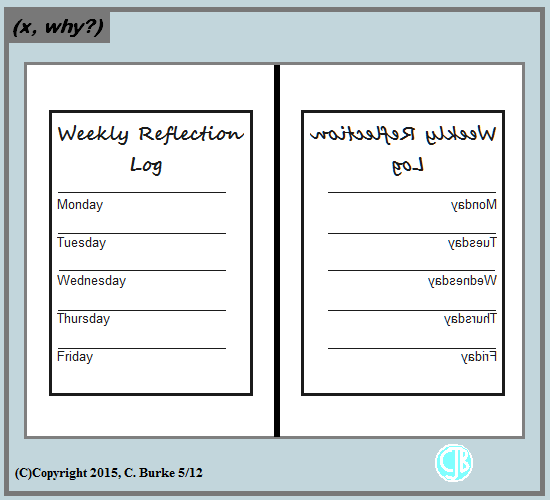The one on the right could be good for left-handed students, right?

But then, maybe it should be on the left.

One of my coworkers uses these. I just started using a weekly one with a weekly question, instead of five separate entries. Starting small, by which I mean, less for them to write because of the bigger timeframe involved.

Come back often for more funny math and geeky comics.## Monday, May 11, 2015

### January 2015 Common Core Algebra Regents, Part II

Update: I now have a Common Core Regents Review books available on Amazon.

If you missed the previous post, here is Part I.

The Common Core exams have more open-ended questions, so I will be splitting them up into two posts. Here are (most of) the Part II questions.

25. Ms. Fox asked her class “Is the sum of 4.2 and the (square root of 2) rational or irrational?” Patrick answered that the sum would be irrational. State whether Patrick is correct or incorrect. Justify your reasoning.

4.2 is a rational number and the square root of 2 is an irrational number. The sum of a rational number and an irrational number is always irrational.

The square root of 2 is 1.414213562… which continues forever without repeating. If you add 4.2, you get 5.614213562… which still continues forever without repeating.

EDIT: Patrick was correct.
This needs to be stated or you will lose a point for not answering the question. That said, if you say that he is correct without any justification, you will not get any points.

26. The school newspaper surveyed the student body for an article about club membership. The table below shows the number of students in each grade level who belong to one or more clubs.

If there are 180 students in ninth grade, what percentage of the ninth grade students belong to more than one club?

There are a total of (33+12=) 45 ninth grade students who are in more than one club. Divide 45/180 = .25 and multiply by 100%. The answer is 25%.

27. A function is shown in the table below.

If included in the table, which ordered pair, (-4,1) or (1, -4), would result in a relation that is no longer a function? Explain your answer.

A relation cannot be a function if there are two separate output values for the same input. The relation contains (-4, 2). If you include (-4, 1) then there will be two outputs for the same input.

This is not the case for (1, -4). There is no x value of 1 in the table yet.

28. Subtract 5x2 + 2x – 11 from 3x2 + 8x – 7. Express the result as a trinomial.

The word from tells you that the second polynomial is the top one in the subtraction problem.

The answer is -2x2 + 6x + 4.

29. Solve the equation 4x2 – 12x = 7 algebraically for x.

If you put y = 4x2 – 12x – 7 into your graphing calculator and find the zeroes, you will not get full credit.

Subtract 7 from both sides of the equation and you get

4x2 – 12x – 7 = 0

You could use guess and check to factor the binomial, knowing that the factors of 7 are only 1 and 7, and the factors of 4 are either 1 and 4 or 2 and 2. There aren’t too many options, so it isn’t a bad possibility – as long as the factors are rational.

The other method is to use the quadratic formula.

The two methods are shown below:

Using either method the solution set is {-0.5, 3.5}

30. Graph the following function on the set of axes below.

This is a piecewise function. Literally, it is a piece of one function and a piece of a different function. The inequalities listed are the domains for each portion of it. In other words, which x-values do you need to use for that part of the graph.

Read the piecewise function like this:

F of x equals the absolute value of x WHEN x is greater than or equal to -3 and less than 1. It is equal to 4 WHEN x is greater than or equal to 1 and less than or equal to 8.

You will only use values between -3 and 8. Do not graph any other values.
The output will look like this:

Note that you need to include the open circle and the closed circle because a function cannot have two y values for any x value. The open circle indicates a boundary that is not part of the solution.

31. A gardener is planting two types of trees: Type A is three feet tall and grows at a rate of 15 inches per year. Type B is four feet tall and grows at a rate of 10 inches per year. Algebraically determine exactly how many years it will take for these trees to be the same height.

First, you have to fix your units. The problem uses both feet and inches. You have to convert to one of them. Converting to inches is easier and won’t require decimals.

Three feet is 36 inches. Four feet is 48 inches.
So Type A grows at: y = 36 + 15x
And Type B grows at: y = 48 + 10x

They will meet at 36 + 15x = 48 + 10x. Subtract 10 from both sides.
That gives you 36 + 5x = 48. Subtract 36 from both sides.
That gives you 5x = 12. Divide by 5.
And x = 12/5 years, which is 2.4 years, which is reasonable because plants grow continuously. They don’t just shoot up 10 or 15 inches at the end of the year.

That’s it for now. Questions 32-37 will be in the next installment.

## Friday, May 08, 2015

### Failed Metric Joke

(Click on the comic if you can't see the full image.)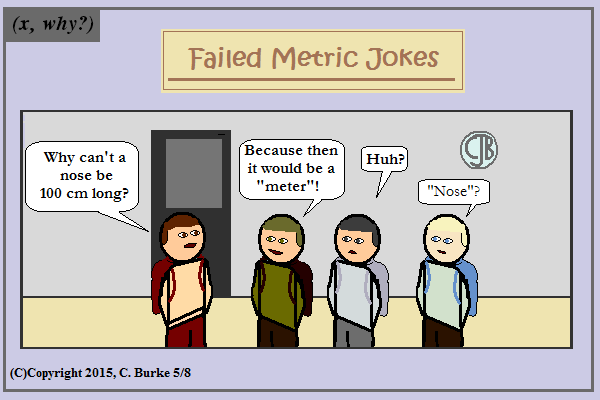Get it? 'Cause 100 would be crazy! or something.

People who advocate a two-coin, 20-cent shift to metric may praise its usefulness but they will be devoid of humor.

Pound for pound, imperial is more creative and whimsical.

Come back often for more funny math and geeky comics.## Thursday, May 07, 2015

### January 2015 Common Core Algebra Regents, Part I

Update: I now have a Common Core Regents Review books available on Amazon.

It's that time of year again. Regents Review is starting, and I realized that I never posted the answers and explanations for the January 2015 Common Core Algebra Regents Exam. Note: the questions can be found online. Answers, too. I tried to explain as well as answer.

So here is Part 1:

1. The owner of a small computer repair business has one employee, who is paid an hourly rate of \$22. The owner estimates his weekly profit using the function P(x) = 8600 - 22x. In this function, x represents the number of ...

(2) The number of hours worked. The hourly rate is \$22, so the employees pay is \$22x, which is subtracted from the profit.

2. Peyton is a sprinter who can run the 40-yard dash in 4.5 seconds. He converts his speed into miles per hour, as shown below.

Which ratio is incorrectly written to convert his speed?

(2) 5280 ft/1 mi. "Feet" needs to be on the bottom to cancel with the previous term. "Mile" needs to be on top in the numerator so that the answer can be expressed in "miles per hour".

3. Which equation has the same solutions as 2x2 + x - 3 = 0?

(4)(2x + 3)(x - 1) = 0. Factor the trinomial into 2 binomials. Or FOIL the binomials until you get the original polynomial.

4. Krystal was given \$3000 when she turned 2 years old. Her parents invested it at a 2% interest rate compounded annually. No deposits or withdrawals were made. Which expression can be used to determine how much money Krystal had in the account when she turned 18?

(1) 3000(1 + 0.02)16. This was a ridiculously easy multiple-choice question. You didn't have to know the rule -- they all have the same format. If you're adding interest, it will be a plus sign. (A minus is for decay.) And if she's 2 years old already, then it will only compound for 16 years.

5. Which table of values represents a linear relationship?

(3). In a linear relationship the rate of change (that is, the slope) is constant. In the table in choice (3), f(x) increases by 2 every time x increases by 1.

6. Which domain would be the most appropriate set to use for a function that predicts the number of household online-devices in terms of the number of people in the household?

(2) Whole numbers. The number of devices cannot be negative or a fraction. Irrational is just silly. Note that "counting numbers" isn't a choice because the number of devices could be zero.

7. The inequality 7 - (2/3)x < x - 8 is equivalent to

(1) x > 9. See below.

8. The value in dollars, v(x), of a certain car after x years is represented by the equation v(x) = 25,000(0.86)x. To the nearest dollar, how much more is the car worth after 2 years than after 3 years?

(1)2589. Calculate v(2), you will get 18,490. Calculate v(3), you will get 15,901. Subtract 18,490 - 15,901 = 2,589. Frankly, it should be obvious from the choices, but work it out anyway just to be sure.

9. Which function has the same y-intercept as the graph below? [Graph omitted]

(4) The y-intercept of the graph is -3. If you switch the choices into slope-intercept form, you will see that only (4) has an intercept of -3.

10. Fred is given a rectangular piece of paper. If the length of Fred’s piece of paper is represented by 2x - 6 and the width is represented by 3x - 5, then the paper has a total area represented by

(2) 6x2 - 28x + 30. Multiply the two binomials to find the Area. Note that -5 times -6 is +30, and only one choice ends in +30!

11. The graph of a linear equation contains the points (3,11) and (-2,1). Which point also lies on the graph?

(4) (2,9). Note that they aren't looking for a midpoint or any other special point. The slope of the line is 10/5 = 2. If that isn't enough to get you the correct choice, then find the equation of the line by figuring out the y-intercept: 11 = (2)(3) + b. Then b = 5, and the equation is y = 2x + 5. The point (2, 9) is a solution to that equation and is on the line.

12. How does the graph of f(x) = 3(x - 2)2 + 1 compare to the graph of g(x) = x2?

(2) The graph of f(x) is narrower than the graph of g(x), and its vertex is moved to the right 2 units and up 1 unit.

Note: you can put both of these into your graphing calculator and look at them. The 3 in f(x) makes that function more narrow. The +1 moves the vertex up. The -2 moves it to the right.

13. Connor wants to attend the town carnival. The price of admission to the carnival is \$4.50, and each ride costs an additional 79 cents. If he can spend at most \$16.00 at the carnival, which inequality can be used to solve for r, the number of rides Connor can go on, and what is the maximum number of rides he can go on?

(3) 4.50 + 0.79r ≤ 16.00; 14 rides. Admission is paid once, no variable. The cost of the rides depends on the number of rides, variable. That means that the answer is either (3) or (4). Substitute 15 for r and you get \$16.35. That is too much money, so it's only 14 rides.

14. Corinne is planning a beach vacation in July and is analyzing the daily high temperatures for her potential destination. She would like to choose a destination with a high median temperature and a small interquartile range. She constructed box plots shown in the diagram below.

Which destination has a median temperature above 80 degrees and the smallest interquartile range?

(4) Pelican Beach. Only two choices have median temperatures (the middle line in the box) above 80. Of those two, (4) has the smaller IQR (the length of the box).

15. Some banks charge a fee on savings accounts that are left inactive for an extended period of time. The equation y = 5000(0.98)x represents the value, y, of one account that was left inactive for a period of x years.
What is the y-intercept of this equation and what does it represent?

(3) 5000, the amount of money in the account initially. The y-intercept is the value when x = 0, which is \$5,000. That is the initial amount of money before any fees are applied. After x years, the balance will be smaller.

16. The equation for the volume of a cylinder is V = πr2h. The positive value of r, in terms of h and V, is

(1). See below.

17. Which equation has the same solutions as x2 + 6x - 7 = 0?

(4) (x + 3)2 = 16. See below

18. Two functions, y = |x - 3| and 3x + 3y = 27, are graphed on the same set of axes. Which statement is true about the solution to the system of equations?

(3) (6,3) is the solution to the system because it satisfies both equations. You could solve the system or you could work backward from the answers. However, there is a much simpler way. Notice that choice (3) is the only one that uses the phrase satisfies both equations. A solution to a system MUST satisfy both equations.

19. Miriam and Jessica are growing bacteria in a laboratory. Miriam uses the growth function f(t) = n2t while Jessica uses the function g(t) = n4t, where n represents the initial number of bacteria and t is the time, in hours. If Miriam starts with 16 bacteria, how many bacteria should Jessica start with to achieve the same growth over time?

(4) 4. Leave off the t for a moment. For what value of n is n4 = 162 = 256? 44 = 256.
Likewise, 162 = (42)2 = 42 X 2 = 44.

20. If a sequence is defined recursively by f(0) = 2 and f(n + 1) = -2f(n) + 3 for n ≥ 0, then f(2) is equal to

(3) 5.
f(0) = 2.
f(1) = -2(f(0)) + 3 = -2(2) + 3 = -4 + 3 = -1.
f(2) = -2(f(1)) + 3 = -2(-1) + 3 = 2 + 3 = 5.

21. An astronaut drops a rock off the edge of a cliff on the Moon. The distance, d(t), in meters, the rock travels after t seconds can be modeled by the function d(t) 0.8t2. What is the average speed, in meters per second, of the rock between 5 and 10 seconds after it was dropped?

(1) 12. The distance traveled in 5 seconds is 0.8(5)2 = 20 feet. The distance traveled in 10 seconds is 0.8(10)2 = 80 feet.
Use the slope formula: (80 - 20)/(10 - 5) = 60/5 = 12.

22. When factored completely, the expression p4 - 81 is equivalent to

(3) (p2 + 9)(p + 3)(p - 3). Difference of Squares Rule gives you (p2 + 9)(p2 - 9). You cannot factor the first term further, but you can factor the second term using the Difference of Squares Rule a second time.

23. In 2013, the United States Postal Service charged \$0.46 to mail a letter weighing up to 1 oz. and \$0.20 per ounce for each additional ounce. Which function would determine the cost, in dollars, c(z), of mailing a letter weighing z ounces where z is an integer greater than 1?

(4) c(z) = 0.20(z - 1) + 0.46. Twenty cents per ounce, variable. Forty-six cents for the first ounce, constant. Subtract 1 from z because the additional postage only applies after the first ounce. In other words, a one-ounce letter would only cost 46 cents.

24. A polynomial function contains the factors x, x - 2, and x = 5. Which graph(s) below could represent the graph of this function?

(1) I, only. First of all, you could put this in the calculator and look at it. Second, you can see that it MUST have three zeroes and that those will be when x = 0, x = 2 and x = -5. That matches the first graph. The third graph is the reverse, which is incorrect. The middle graph is a parabola with only 2 roots.

## Wednesday, May 06, 2015

(Click on the comic if you can't see the full image.)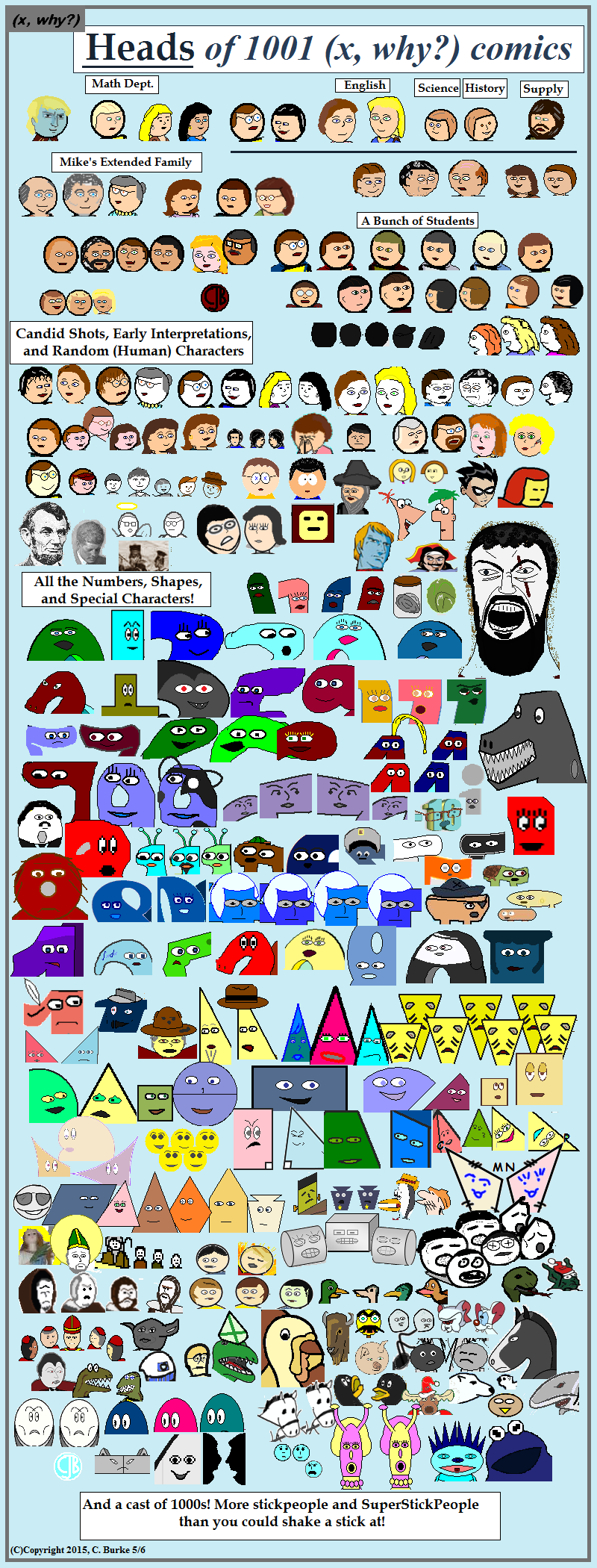Or 'Faces' when I wasn't sure what was actually the head.

I actually started working on this two weeks earlier and tried to sneak work in whenever I could. Even at that, I only made it through about 850 comics. Lots of the heads were repeats, although some had changed.

I already planned on leaving out countless numbers of nameless stick figures (and a few named ones) before realizing that I had to leave them all out along with all the photo characters. Most of those were plushies and I was only going to include the Star Trek ones -- how many trademarks did I want to possibly infringe upon in one comic?

The kicker of it is that I actually wanted to identify as many of the characters as I could, sort of a Who's Who, but I had neither room nor time. (And in some cases, names, but I could work around that.)

Happy to start my second thousand comics. I hope to finish them in fewer than seven and a half years.

Come back often for more funny math and geeky comics.## Friday, May 01, 2015

### More Math and Coffee (Number 1000!)

(Click on the comic if you can't see the full image.)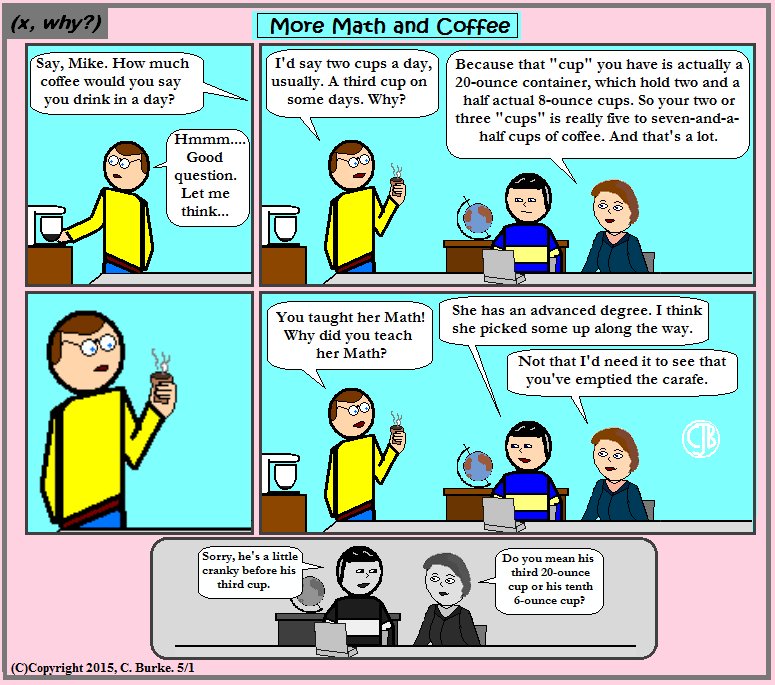For the thousandth time, it's still only two cups. Or three.

This is why the Imperial system is superior to Metric. I can happily drink my two or three cups, but you'll know that you're consuming hundreds, even thousands of milliliters!

Happy 1000th Comic. I hope no one was expecting A (x, why?) Wedding or something like that.

Come back often for more funny math and geeky comics.